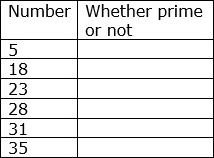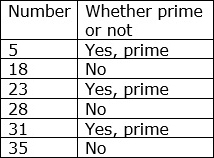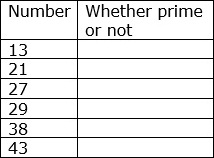# Prime Numbers

### Prime numbers

A number is a prime number if its only factors are 1 and itself.

7 is a prime number. Its factors are 1 and 7 itself

Some examples of prime numbers are 2, 3, 5, 7, 11, 13 and so on.

2 is the only even prime number. All other prime numbers are odd numbers

### Composite numbers

If a number has three or more factors, it is a composite number. A number which has factors in addition to one and itself is called a composite number.

6 is a composite number. It has four factors; 1, 2, 3 and 6

For example: 4, 6, 8, 9, 10, 12,...are examples of some composite numbers

All even numbers except 2 are composite numbers.

Neither Prime nor Composite

0 and 1 are neither prime numbers nor composite numbers.

State whether each number in table below is prime or not.### Solution

Step 1:Step 2:

Of the given numbers 18, 28 and 35 have three or more factors. So they are not prime but composite numbers.

Step 3:

The numbers 5, 23 and 31 have only 1 and themselves as factors. So these numbers are prime numbers.

State whether each number in table below is prime or not.### Solution

Step 1:Step 2:

Of the given numbers 21, 27 and 38 have three or more factors. So they are not prime but composite numbers.

Step 3:

The numbers 13, 29 and 43 have only 1 and themselves as factors. So these numbers are prime numbers.